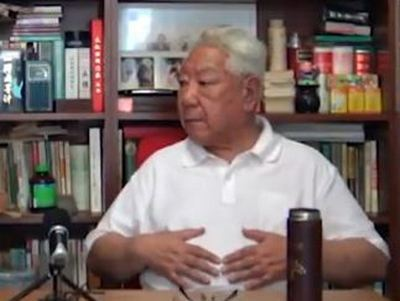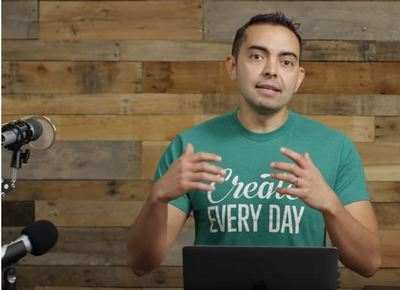Like   Tweet   Pin   +1   in/* styles */ Performance + Wellbeing Stories, Tips and Resources   ||   Issue #30 September 2020
 table div table+table+table div table{width:100%;padding:0}table div table+table+table div table img{width:96.23%;padding:0;float:none}table div table+table+table div table td{width:100%;padding:0 1.88% 18px}/* styles */Welcome to September's issue of Good Chi News. There's a short article on how I'm coping with the pandemic virus restrictions, a Tai Chi tip, and two short YouTube clips on the difference between Tai Chi and Chi Kung and the History of Wu Style Tai Chi.

You'll also find a new feature - my favourite Tai Chi and Chi Kung books - and there's a YouTube clip on how to set up a podcast. Plus our regular ancient Chinese quote.

Regards
Chris Bennett
Chris Chi

 table div table+table+table+table+table div table{width:100%;padding:0}table div table+table+table+table+table div table img{width:96.23%;padding:0;float:none}table div table+table+table+table+table div table td{width:100%;padding:0 1.88% 18px}/* styles */## Coping with the Pandemic Virus RestrictionsI haven't had any face-to-face training sessions this year because of the restrictions due to the Covid-19 pandemic.

But I've made sure I've kept busy by working on creating online programs and working on the fourth draft of my memoir.

The three key things I have found that have helped me to cope with all the negativity from the pandemic are:

1. Regular Tai Chi training
2. Having the same routine as I did before the virus hit
3. Adapting work to my home environment
 1 Regular Tai Chi training
 2 Having the same routine as I did before the virus hit
 3 Adapting work to my home environment

In a way, I'm following the advice of an ancient Chinese quote - 'Invest in Loss'.

Image courtesy of Eric Muhr via Unsplash

 table div table+table+table+table+table+table+table+table div table{width:100%;padding:0}table div table+table+table+table+table+table+table+table div table img{width:96.23%;padding:0;float:none}table div table+table+table+table+table+table+table+table div table td{width:100%;padding:0 1.88% 18px}/* styles */## Tai Chi Tip

 /* styles */ Breath in a smooth, slow and deep manner.
 table div table+table+table+table+table+table+table+table+table+table+table div table{width:100%;padding:0}table div table+table+table+table+table+table+table+table+table+table+table div table img{width:96.23%;padding:0;float:none}table div table+table+table+table+table+table+table+table+table+table+table div table td{width:100%;padding:0 1.88% 18px}/* styles */## Difference Between Tai Chi and Chi KungI'm sometimes asked what the difference is between Chi Kung and Tai Chi.

Here's a clear and basic explanation for beginners on the difference between Tai Chi and Chi Kung from Jon of Mastering Life Force.

 table div table+table+table+table+table+table+table+table+table+table+table+table+table+table div table{width:100%;padding:0}table div table+table+table+table+table+table+table+table+table+table+table+table+table+table div table img{width:96.23%;padding:0;float:none}table div table+table+table+table+table+table+table+table+table+table+table+table+table+table div table td{width:100%;padding:0 1.88% 18px}/* styles */## History of Wu Style Tai ChiHere's an informative clip on the history of Wu Style Tai Chi.

The interviewee is Mai Hai Long and he gives a very interesting interview on the history of Wu Style Tai Chi.

My background is in learning and teaching this style of Tai Chi for over thirty years

 table div table+table+table+table+table+table+table+table+table+table+table+table+table+table+table+table+table div table{width:100%;padding:0}table div table+table+table+table+table+table+table+table+table+table+table+table+table+table+table+table+table div table img{width:96.23%;padding:0;float:none}table div table+table+table+table+table+table+table+table+table+table+table+table+table+table+table+table+table div table td{width:100%;padding:0 1.88% 18px}/* styles */## My Library BooksThis is a new feature for Good Chi News about helpful books to read on Tai Chi and Chi Kung.

Each issue I'll recommend one book from my library that I feel would be worth reading. The books will range from basic to advanced levels.

Here's the first book I recommend. It's a Chi Kung book called 'The Way of Energy" by Master Lam Kam Chuen.(1991 - Gaia Books Ltd). It's available from several online bookstores, including Book Depository.

Image courtesy of Cesar Viteri via Unsplash

 table div table+table+table+table+table+table+table+table+table+table+table+table+table+table+table+table+table+table+table+table div table{width:100%;padding:0}table div table+table+table+table+table+table+table+table+table+table+table+table+table+table+table+table+table+table+table+table div table img{width:96.23%;padding:0;float:none}table div table+table+table+table+table+table+table+table+table+table+table+table+table+table+table+table+table+table+table+table div table td{width:100%;padding:0 1.88% 18px}/* styles */## Chris Chi NewsI discovered this clip by Pat Flynn on how to set up a podcast, while researching my planned podcast

It focuses on the equipment and software you'll need and it's ideal if you want to set up one for yourself or your organisation.

This basic information is what I need as a beginner.

 table div table+table+table+table+table+table+table+table+table+table+table+table+table+table+table+table+table+table+table+table+table+table+table div table{width:100%;padding:0}table div table+table+table+table+table+table+table+table+table+table+table+table+table+table+table+table+table+table+table+table+table+table+table div table img{width:96.23%;padding:0;float:none}table div table+table+table+table+table+table+table+table+table+table+table+table+table+table+table+table+table+table+table+table+table+table+table div table td{width:100%;padding:0 1.88% 18px}/* styles */table div table+table+table+table+table+table+table+table+table+table+table+table+table+table+table+table+table+table+table+table+table+table+table+table+table div table{width:100%;padding:0}table div table+table+table+table+table+table+table+table+table+table+table+table+table+table+table+table+table+table+table+table+table+table+table+table+table div table img{width:96.23%;padding:0;float:none}table div table+table+table+table+table+table+table+table+table+table+table+table+table+table+table+table+table+table+table+table+table+table+table+table+table div table td{width:100%;padding:0 1.88% 18px}/* styles *//* styles */
 table div table+table+table+table+table+table+table+table+table+table+table+table+table+table+table+table+table+table+table+table+table+table+table+table+table+table+table div table{width:100%;padding:0}table div table+table+table+table+table+table+table+table+table+table+table+table+table+table+table+table+table+table+table+table+table+table+table+table+table+table+table div table img{width:96.23%;padding:0;float:none}table div table+table+table+table+table+table+table+table+table+table+table+table+table+table+table+table+table+table+table+table+table+table+table+table+table+table+table div table td{width:100%;padding:0 1.88% 18px}/* styles *//* styles */